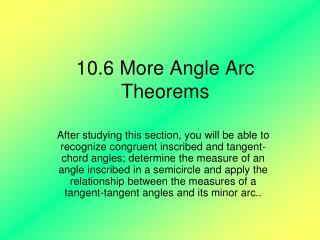DownloadDownload Presentation10.6 More Angle Arc Theorems

# 10.6 More Angle Arc Theorems

Download Presentation## 10.6 More Angle Arc Theorems

- - - - - - - - - - - - - - - - - - - - - - - - - - - E N D - - - - - - - - - - - - - - - - - - - - - - - - - - -
##### Presentation Transcript

1. 10.6 More Angle Arc Theorems After studying this section, you will be able to recognize congruent inscribed and tangent-chord angles; determine the measure of an angle inscribed in a semicircle and apply the relationship between the measures of a tangent-tangent angles and its minor arc..

2. A C B O O E D Theorem If two inscribed or tangent chord angles intercept the same arc, then they are congruent. Theorem If two inscribed or tangent-chord angles intercept congruent arcs, then they are congruent.

3. Theorem The sum of the measures of a tangent- tangent angle and its minor arc is 180. Minor arc DE = 130 find the measure of angle F. D O 130 F E

4. Example 1 Given circle O N Conclusions Triangle LVE is similar to triangle NSE L E O S V

5. Example 2 In circle O, segment BC is a diameter and the radius of the circle is 20.5 mm. Chord AC has a length of 40 mm. Find AB. A B O C

6. Example 3 B A Given: circle O with tangent at B D C O Prove:

7. Summary Summarize what you have learned in this lesson. Homework: worksheet